Question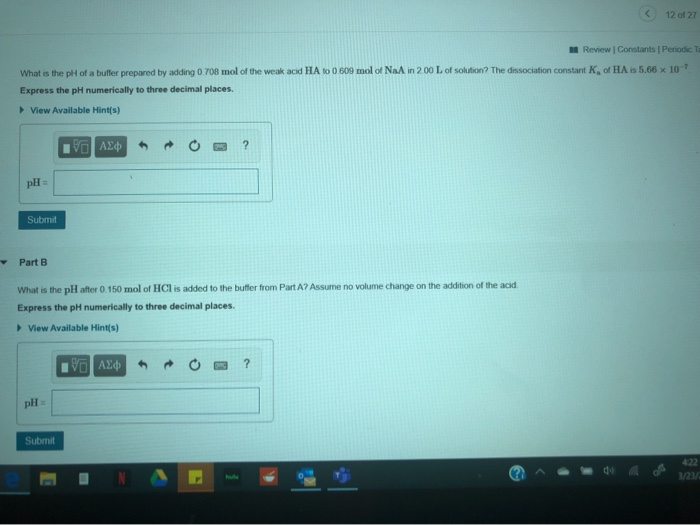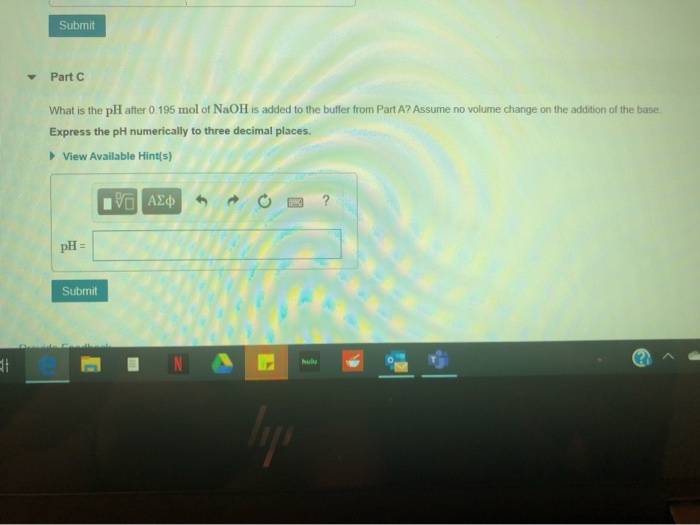Part -A

pH = 6.182

part - B

pH = 5.976

Part - C

pH = 6.442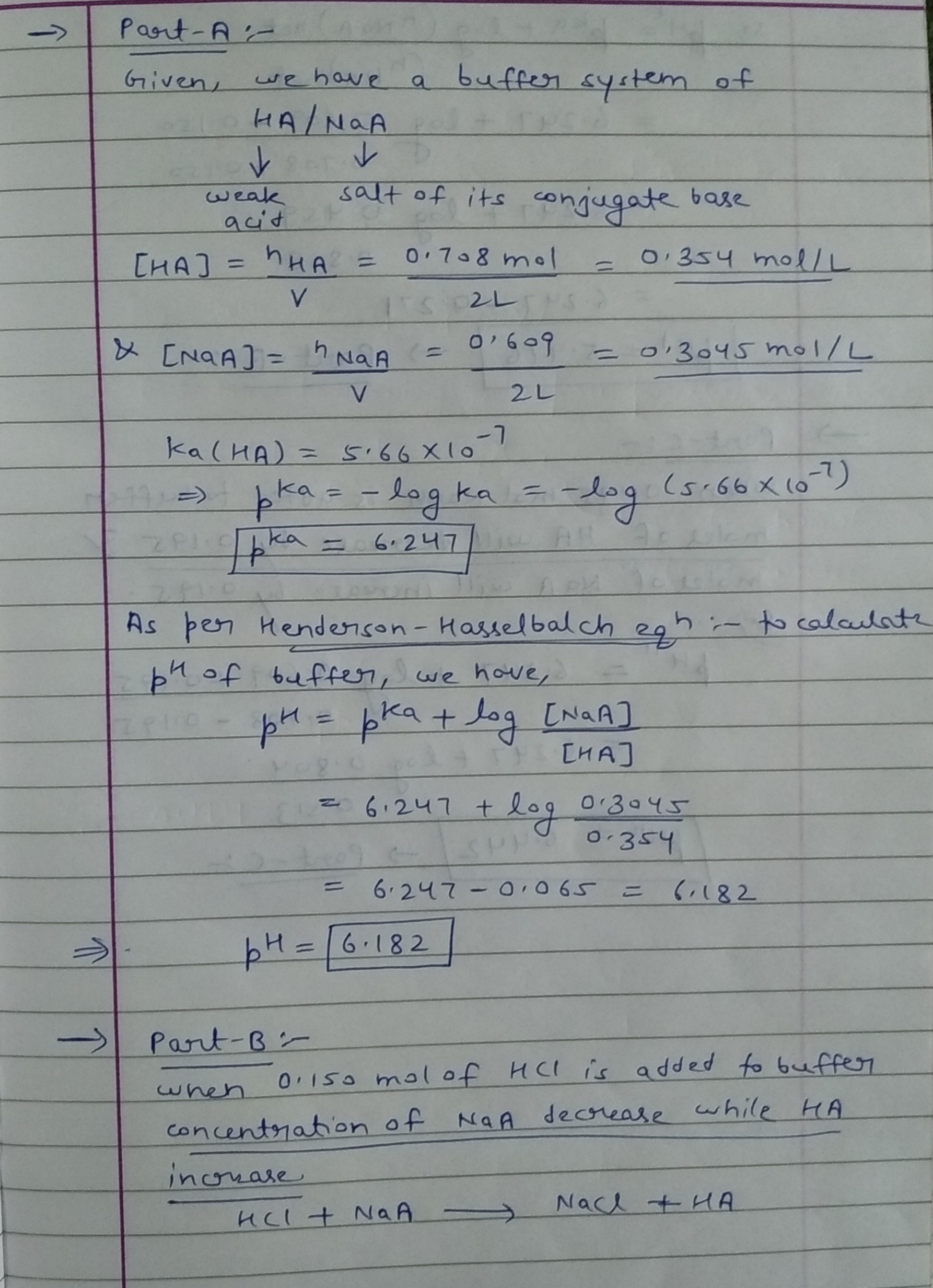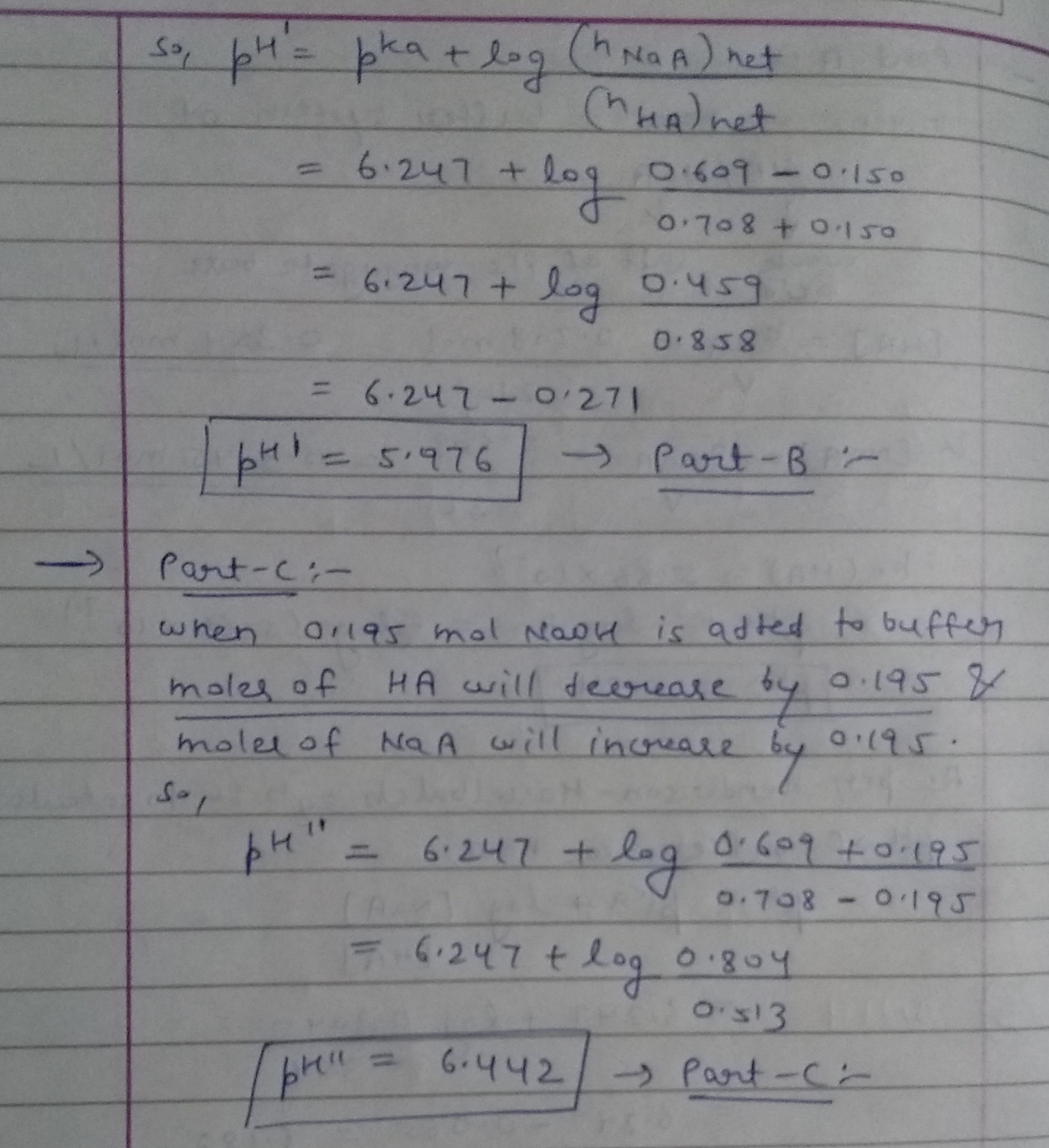#### Earn Coins

Coins can be redeemed for fabulous gifts.

Similar Homework Help Questions
• ### Part A - 7 What is the pH of a buffer prepared by adding 0.809 mol...Part A - 7 What is the pH of a buffer prepared by adding 0.809 mol of the weak acid HA to 0.507 mol of NaA in 2.00 L of solution? The dissociation constant K, of HA is 5.66 x 10 Express the pH numerically to three decimal places. View Available Hint(s) Ivo AED ? pH = Submit Part B What is the pH after 0.150 mol of HCl is added to the buffer from Part A? Assume no volume...

• ### ± pH Changes in Buffers Part A What is the pH of a buffer prepared by...

± pH Changes in Buffers Part A What is the pH of a buffer prepared by adding 0.708 mol of the weak acid HA to 0.608 mol of NaA in 2.00 L of solution? The dissociation constant Ka of HA is 5.66×10−7. Express the pH numerically to three decimal places. Part B What is the pH after 0.150 mol of HCl is added to the buffer from Part A? Assume no volume change on the addition of the acid. Express...

• ### M Review | Constants | Periodic Table - Part A When a solution contains a weak...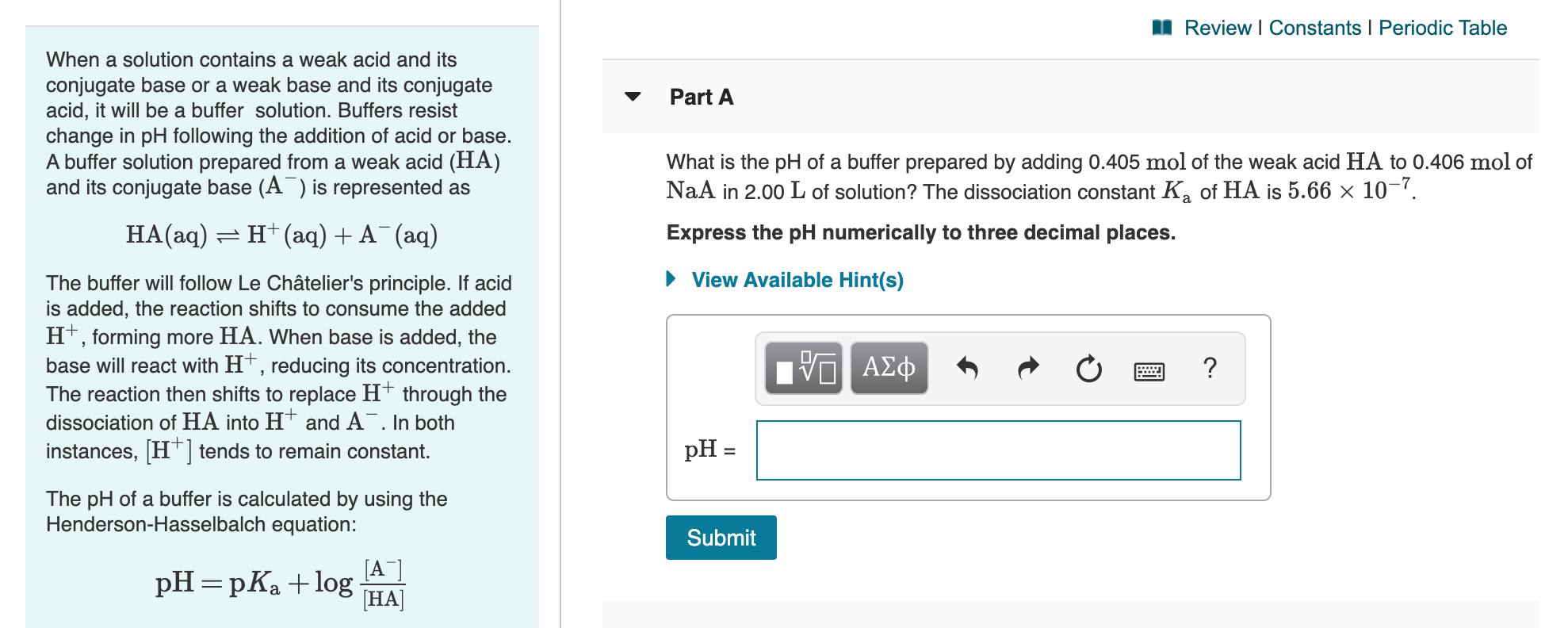M Review | Constants | Periodic Table - Part A When a solution contains a weak acid and its conjugate base or a weak base and its conjugate acid, it will be a buffer solution. Buffers resist change in pH following the addition of acid or base. A buffer solution prepared from a weak acid (HA) and its conjugate base (A) is represented as What is the pH of a buffer prepared by adding 0.405 mol of the weak acid...

• ### What is the pH after 0.150 mol of HCl is added to the buffer from Part...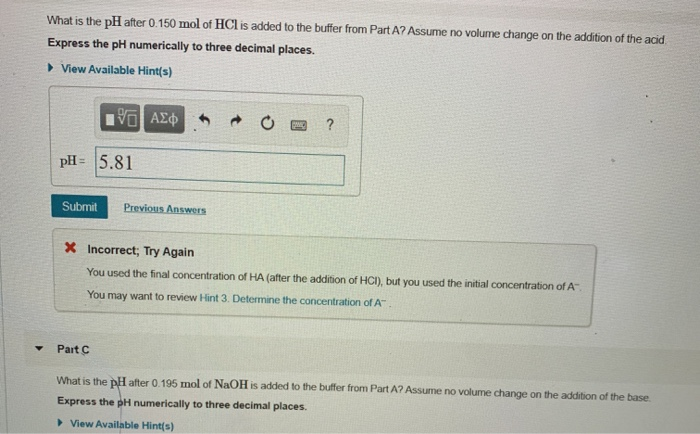What is the pH after 0.150 mol of HCl is added to the buffer from Part A? Assume no volume change on the addition of the acid Express the pH numerically to three decimal places. ► View Available Hint(s) ROO? V AE pH= 5.81 Submit Previous Answers X Incorrect; Try Again You used the final concentration of HA (after the addition of HCI), but you used the initial concentration of A You may want to review Hint 3. Determine the...

• ### Part A What is the pH of a buffer prepared by adding 0.708 mol of the weak acid HA to 0.406 mol of NaA in 2.00 Lof sol...

Part A What is the pH of a buffer prepared by adding 0.708 mol of the weak acid HA to 0.406 mol of NaA in 2.00 Lof solution? The dissociation constant Ka of HA is 5.66×10−7. Express the pH numerically to three decimal places. Part B What is the pH after 0.150 mol of HCl is added to the buffer from Part A? Assume no volume change on the addition of the acid. Express the pH numerically to three decimal...

• ### Part A What is the pH of a buffer prepared by adding 0.506 mol of the weak acid HA to 0.608 mol of NaA in 2.00 L of so...

Part A What is the pH of a buffer prepared by adding 0.506 mol of the weak acid HA to 0.608 mol of NaA in 2.00 L of solution? The dissociation constant Ka of HA is 5.66×10−7. Express the pH numerically to three decimal places. pH = SubmitHintsMy AnswersGive UpReview Part Part B What is the pH after 0.150 mol of HCl is added to the buffer from Part A? Assume no volume change on the addition of the acid....

• ### Part A: What is the pH of a buffer prepared by adding 0.708 mol of the...

Part A: What is the pH of a buffer prepared by adding 0.708 mol of the weak acid HA to 0.406 mol of NaA in 2.00 L of solution? The dissociation constant Ka of HA is 5.66×10−7. Part B:What is the pH after 0.150 mol of HCl is added to the buffer from Part A? Assume no volume change on the addition of the acid. Part C: What is the pH after 0.195 mol of NaOH is added to the...

• ### Review Constants Periodic Table Iso results in a pH shift of the system. is the presence...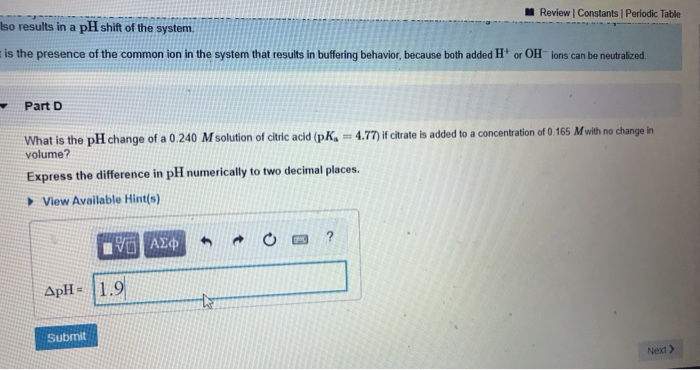Review Constants Periodic Table Iso results in a pH shift of the system. is the presence of the common ion in the system that results in buffering behavior, because both added H or OH ions can be neutralized. Part D What is the pH change of a 0.240 M solution of citric acid (p =4.77) if citrate is added to a concentration of 0.165 M with no change in volume? Express the difference in pH numerically to two decimal places....

• ### What is the pH of a buffer prepared by adding 0.506 mol of the weak acid HA to 0.305 mol of NaA in 2.00  L of solu...

What is the pH of a buffer prepared by adding 0.506 mol of the weak acid HA to 0.305 mol of NaA in 2.00  L of solution? The dissociation constant Ka of HA is 5.66*10^-7. pH = __________ What is the \rm pH after 0.150 mol of \rm HCl is added to the buffer from Part A? Assume no volumechange on the addition of the acid. pH = _____________ What is the \rm pH after 0.195 mol of \rm NaOH...

• ### Constants 1 Per A certain weak acid, HA, with a K value of 5.61 x 10-6,...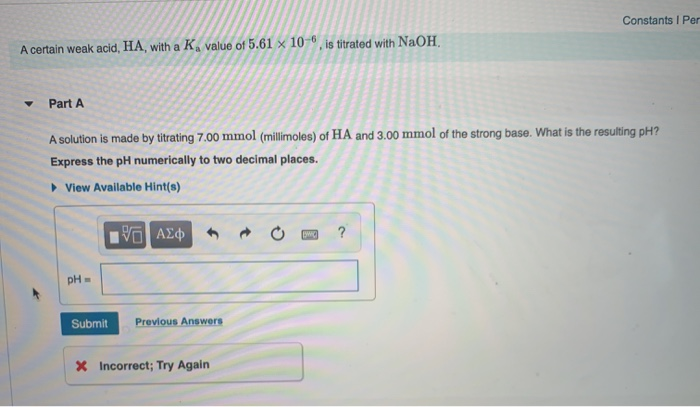Constants 1 Per A certain weak acid, HA, with a K value of 5.61 x 10-6, is titrated with NaOH. Part A A solution is made by titrating 7.00 mmol (millimoles) of HA and 3.00 mmol of the strong base. What is the resulting pH? Express the pH numerically to two decimal places. View Available Hint(s) IVO AQ R O ? pH в Submit Previous Answers X Incorrect; Try Again Part B More strong base is added until the equivalence...